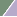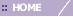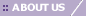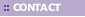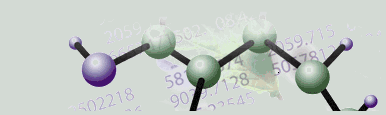# Distance Metrics Overview

Overview

Distance Measurements Between Data Points

This parameter specifies how the distance between data points in the clustering input is measured. The options are:

• Euclidean: Use the standard Euclidean (as-the-crow-flies) distance.

• Euclidean Squared: Use the Euclidean squared distance in cases where you would use regular Euclidean distance in Jarvis-Patrick or K-Means clustering.

• Manhattan: Use the Manhattan (city-block) distance.

• Pearson Correlation: Use the Pearson Correlation coefficient to cluster together genes or samples with similar behavior; genes or samples with opposite behavior are assigned to different clusters.

• Pearson Squared: Use the squared Pearson Correlation coefficient to cluster together genes with similar or opposite behaviors (i.e. genes that are highly correlated and those that are highly anti-correlated are clustered together).

• Chebychev: Use Chebychev distance to cluster together genes that do not show dramatic expression differences in any samples; genes with a large expression difference in at least one sample are assigned to different clusters.

• Spearman: Use Spearman Correlation to cluster together genes whose expression profiles have similar shapes or show similar general trends (e.g. increasing expression with time), but whose expression levels may be very different.

Distance Measurements Between Clusters

This parameter specifies how the distance between clusters is measured. The options are:

• Average Linkage: The distance between two clusters is the average of the distances between all the points in those clusters.

• Single Linkage: The distance between two clusters is the distance between the nearest neighbors in those clusters.

• Complete Linkage: The distance between two clusters is the distance between the furthest points in those clusters.

Related Topics:

Overview of K-Means Clustering

Overview of Jarvis-Patrick Clustering

Overview of Agglomerative Hierarchical Clustering